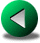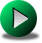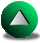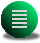Previous: Conservative Properties of the
Next: Conclusions
Up: Testing the Different Numerical

# The Munk Problem in a Square Domain

In this section, we compare the models using a second nonlinear problem, namely the single gyre Munk problem. With a constant wind, the sphericity and rotation of the earth yield a strong return flow along the western wall. The wind forcing is given by the stress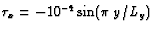m2s-2 and. The remaining model parameters are identical to those of the previous section. The energy put in the ocean by the winds is dissipated mainly in a viscous layer along the boundary because of the strong return flow there. The eddy-viscosity,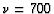m2s-1, is constant over the whole domain. We use the free-slip boundary condition. A strong recirculation forms in the northwestern part of the domain, evidence of the nonlinear effects in the solution. Under free-slip, the solution is very sensitive to the shape of the boundaries and to the value of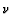. We hope to shed some light on the sensitivity of the FD models to steps occurring along the boundaries, as FD models generally do not work well in irregular geometries. Furthermore, because of the sensitivity of the solution to, we expect to better observe the dissipative nature of FE models.

For the C-grid FD model, Adcroft and Marshall (1998), hereafter AM, performed the same test-case for somewhat different model parameters. An important finding in this study is that the C-grid model is very sensitive to the presence of steps, to the point that simulations run in a rotated square basin with respect to the grid yield very different results compared to the non-rotated simulation. This sensitivity could greatly be reduced if the conventional five-point Laplacian in the viscous tensor is replaced by a discretized vorticity-divergence form. The two tensor formulations are equivalent in a non-rotated basin, but are different in presence of steps. Around steps, the vorticity-divergence formulation tends to accelerate the fluid parcels compared to the conventional stress formulation. Their findings suggest that free-slip circulations can be made independent of the way the coastline is discretized. We shall return to this issue in the next chapter.

We consider the solution from the 4th order A-grid model. When running the nonlinear version of this model with free-slip boundary conditions, we noted that having 4th order accuracy extended up to the boundaries has some positive influence on the stability of the overall model. Figure 3.13 shows that large spurious numerical modes are present for O-FDM4, whereas there are no visible spurious modes for R-FDM4. We also consider the same experiment in a rotated basin with respect to the grid, following AM. Strong numerical noise again occurs for O-FDM4 (Fig. 3.14). The model remains however stable and relatively noise-free when the 4th order extends up to the walls, although the total kinetic energy is less than that for the non-rotated basin experiment. Moreover, the overall circulation looks very similar to that observed by AM with the C-grid and conventional viscous stress tensor in rotated basins. The C-grid solutions tend to be less noisy, though. These two observations (numerical noise and lower energetic level) demonstrate that the 4th order formulation is very sensitive to the presence of steps when the free-slip boundary condition is used. We did not test whether the vorticity-divergence form of the stress tensor has the same positive influence for R-FDM4, as it does in the case of the C-grid. Chapter 4 will be specifically dedicated to a thorough study of the issue of FD discretizations and advective and stress tensor formulations in rotated basins.We compare now the FE models to the solution given by the C-grid FD model for a non-rotated basin. All FE models tend to show a kinetic energy value well below the FD model during spin-up (Fig. 3.15). The circulation also proves to be weaker in magnitude for the FE models when compared to the FD model circulation (Fig. 3.16), showing the dissipative nature of FE models. We discuss some of the reason for this behavior. For the HT model, increasing the resolution did not improve the solution (not shown). There is therefore some sort of zero truncation order error in this model. This may arise from the discretization error of the nonlinear terms in the momentum equations due to the discontinuous linear form of the velocity basis functions. The PZM model may be dissipative because of the use of averaged values at triangle centroids in the computation of fluxes. The LLS is dissipative because of the dissipative nature of the low order Kriging method used in the time semi-Lagrangian discretization (see Section 2.3 for more details). We do not expect to see any improvement with increased resolution for this model because higher resolution means smaller time-steps, and therefore, a larger number of interpolation operations.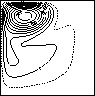C-grid FDlumped LWdelumped LWHTPZMLLS

For the LW model, the mass equation is not solved independently for the elevation but is mixed with a wave equation (Eq. 2.36). In theory, both equations should be satisfied independently. However, since both equations are mixed together, neither is solved exactly and this may influence the overall oceanic circulation. The wave equation tends to transfer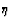, equivalent to the mass, through the whole domain by means of gravity waves. This process may upset the local geostrophic balance by transferring the mass through the streamlines. This process is equivalent to having a dissipation term in the mass equation. To illustrate this, we vary the value for, the free parameter appearing in the wave-mass equation for the wind-driven single gyre Munk problem. Figure 3.17 shows that the kinetic energy for a single gyre wind forcing at the end of the simulation varies significantly with the value of(not to be confused with the wind stress; see Section 2.3 for details). In the limit, which corresponds to satisfying the local mass balance, the results are very similar to the ones obtained using the FD method. However, as we noted earlier the model can be unstable for large values offor certain applications (Section 3.3). A good compromise is found by experimenting with different values ofand is therefore very application dependent.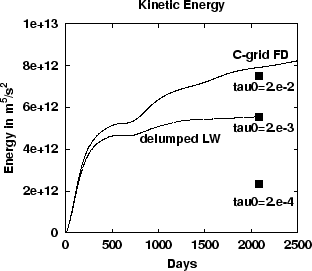For the discontinuous SE model, we consider the Munk problem for two values of the eddy-viscosity. We retained for comparison the C-grid FD model and the delumped LW FE model at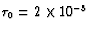s-1, which gives better results than the lumped version. The SE model is run at nc=5 on a 56 triangle mesh. We compared results from the FD, SE and FE models for two values of the viscosity coefficient. For the high viscosity case (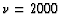m2s-1, Fig.3.18) the models perform similarly, with the FE model showing smaller undershoots. The SE and FD kinetic energy curves are indistinguishable. For the SE model, Figure 3.19 gives the contours of the elevation at the end of the simulation. No discontinuities are visible, despite the fact that the solution is discontinuous by definition. A larger discrepancy is observable for a lower viscosity case (m2s-1, Fig.3.20) between the FE model and the FD and SE models, due to the dissipative nature of the LW model. At the end of the 6 year simulation, there is a 5% difference between the kinetic energy for the FD and SE models. This is an evidence that the SE model lacks resolution in certain parts of the domain, as some discontinuities are now visible in the elevation field (Fig.3.21).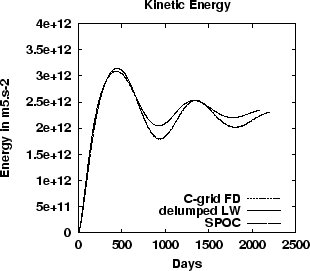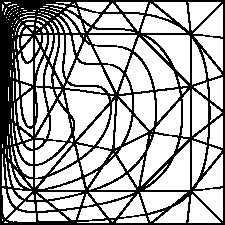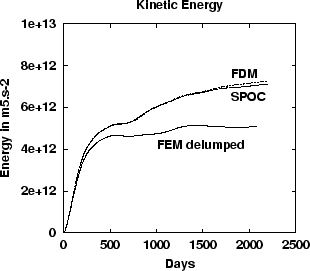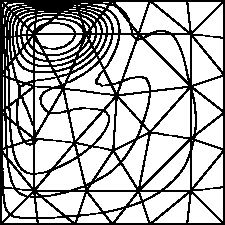We now examine the accuracy and cost of the FD and SE models. We have discarded the solutions obtained by all FE models because of their over-dissipative behavior. As an indicator of the accuracy, we use the kinetic energy of the basin. Since the solution of this test problem is nonlinear, a reference solution is obtained by running the spectral model for 6 years from rest with nc=7 and a mesh of 132 nodes. The error is then defined as the difference between the value of the kinetic energy obtained by one model after a 6 year run and that of the reference solution. The normalized error is computed by dividing the error by the value of the kinetic energy found in the reference solution. Figures 3.22 and 3.23 show the convergence of the error with resolution and CPU cost respectively. The fact that the finite difference results give close to second order accuracy suggests the reference solution is an accurate approximation of the true solution. These two figures confirm in general the behavior inferred from the linear test case. The convergence with resolution and CPU time is faster with higher order methods. However, the fact that there is a cross-over point indicates that below a certain resolution (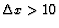km), the FD model is more accurate for the same cost. At the cross-over point the error in kinetic energy is less than 1%. Therefore, the SE model is more cost-effective than the FD model in a range of resolution for which the overall error is already below 1%.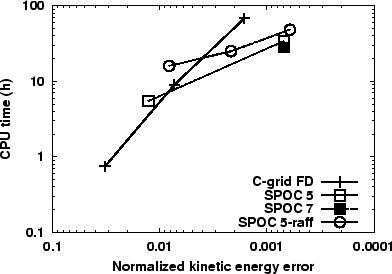It is also of interest to investigate the cost-effectiveness of the adaptive refinement strategy developed in Section 2.4.3 for the SE model. Since this allows for variable resolution in space and time, it may prove more effective than having a fixed and rather uniform mesh in time. We use the refinement parameters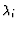and ncheck given in Table 2.2 and we test the SE model for the Munk problem withm2s-1 for three values for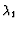(0.3, 0.2 and 0.1), which controls the maximum discontinuity allowable between two elements. We obtain the circulation patterns of Figure 3.24 (middle panels) and meshes (top panels) at the end of the 6 year simulation. The time evolution of the number of elements for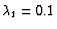(the smallest value used) shows that part of the refinement goes into following the Kelvin waves at the beginning of the simulation, which require more resolution along the boundaries (Fig. 3.24, bottom panels). When the Kelvin adjustment process weakens, a derefinement process occurs along the eastern and southern boundaries leaving higher resolution regions along the strong western return flow. Asdecreases, the refined triangles get smaller and smaller, and the total number of elements at the end of the simulation increases slightly. The isolines of the elevation field are smoother than those of Figure 3.21, for which a fixed and rather uniform 56-triangle mesh was used for the SE model. Therefore, the 56-triangle mesh is too coarse to model this particular Munk problem withm2s-1. We also note that the isolines are slightly smoother asdecreases. The convergence rate of the error in kinetic energy with resolution is better than the SE model at nc=5. However, the accuracy-to-cost convergence is not as good with the cross-over point of the FD model being at a higher accuracy level. This may be due to the fact that the refinement needed to resolve the Kelvin waves along the boundaries at the beginning of the simulation results in smaller time steps. This failure points also to a need for local time-stepping, although it is not quite clear how to implement such a procedure without loss of accuracy. Of interest is to note that the error in the kinetic energy decreases faster than. For instance, we gain about one order in the the kinetic energy error by decreasingby a factor three. If the SE model were truly of truncation order nc close to the element edges, the kinetic energy error should have decreased by the same factor as. This tends to prove that the errors in the SE model are larger at the boundary between elements where the discontinuities occur. However, these errors do not seem to adversely affect the overall accuracy, possibly because these larger errors are localized to the edges of the elements.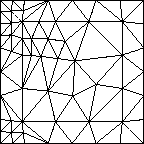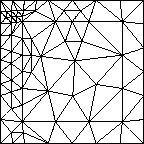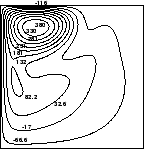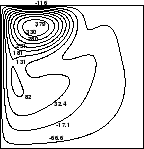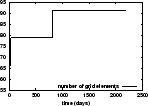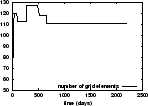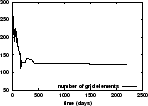a b c

Table 3.3: Convergence order for the different models for the nonlinear Munk problem in a square domain.
 Model convergence order for the error in kinetic energy C-grid FD 2.18 SPOC 5 4.96Previous: Conservative Properties of the
Next: Conclusions
Up: Testing the Different Numerical

Frederic Dupont
2001-09-11